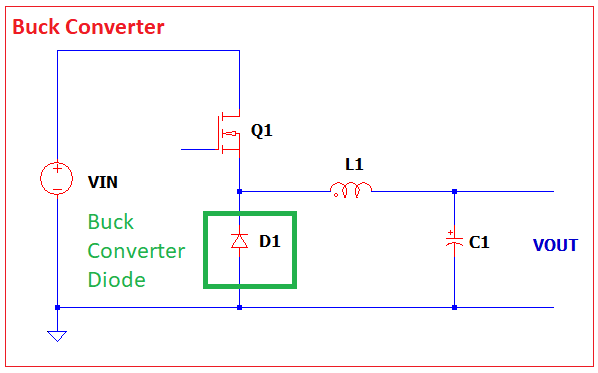# How to Select a Buck Converter Diode

Diode is very important integral part of a buck converter. It is very popular than using a switch in the same location. A diode does not need a control mechanism while a switch needs. A diode is cheaper than a switch considering there is an added control mechanism. Here are the guides on how to select a buck converter diode. If you follow all these, your design will be a rock solid.

# 9 Key Items on How to Select a Buck Converter Diode

## 1. Forward Current Rating

The diode will conduct only when the switch turns off. That is why it is called a catch diode. Thus, the current in the diode is trapezoidal in shape. Refer to below waveform.

From the above waveform, there is only a current when the PWM is at off state. The effective current on the diode must consider the complete switching cycle. Refer to the article Buck Converter Design Tutorial on how the current is derived.

When you already have the current, either in RMS or DC form, you can start looking for a diode from online catalogues like Mouser and Digikey to name few.

For instance, the RMS value you computed from the buck converter diode waveform is 10A, select a diode which can withstand this current plus lot of margin. Setting the current stress level to 60% is a good practice. Thus, for a 10A current, the diode current rating must be at least 17A

## 2. Peak Forward Current

This is also called repetitive peak forward current in some datasheets. This is the maximum current the diode could withstand in a given duration. This is essential in switching converter like the buck converter.

The peak of the current waveform below in green must not exceed the datasheet value.

## 3. Voltage Rating

The next important parameter on how to select a buck converter diode is to ensure the voltage rating is not exceeded.

When the diode is not conducting, the voltage across it is high. In ideal case in a buck converter, the voltage seen by the diode is just the level of VIN. However, switching converter is known to have parasitic elements in which add spike to the diode, therefore adding more margins to the voltage is recommended.

Since the spike factor is unpredictable, in practice doubling the voltage rating of a diode from the ideal voltage is a good start. For instance, the VIN level is 24V, thus select a diode with a voltage rating of at least 48V.

## 4. Forward Voltage

In power electronics, a very small forward voltage drop is highly recommended. The forward voltage is directly proportional to the conduction loss or static power dissipation of the diode. The conduction loss is just the product of forward current (the actual current flowing to the diode) and the forward voltage. In other words, a small forward voltage will result to a small power loss.

In power supply, efficiency is a strict requirement. Thus, a smaller forward voltage drop is ideal as it can give a higher efficiency.

## 5. Power Dissipation Rating or Power Rating

Power rating is the capacity of the diode to handle power dissipation. Power dissipation will result into a heat. Most datasheets provide power rating under nominal ambient temperature which is most of the times 25’C. However, when the operation is higher than this temperature, the power dissipation at the nominal temperature is no longer valid.

The so called de-rated power dissipation is a function of the junction temperature, ambient or case temperature and the thermal resistance.

De-rated Power = (Tjmax – Tambient) / Rthja

Or

De-rated Power = (Tjmax – Tcase) / Rthjc

## 6. Junction Temperature

A diode with a higher junction temperature will tolerate more heat. For instance, a diode with 175’C junction temperature is better than a diode which only allow up to 150’C junction temperature. There is a relationship between a junction temperature and the power dissipated by a diode. A higher junction temperature could allow higher power dissipation on the diode.

## 7. Thermal Resistance

The thermal resistance is needed to compute the de-rated power of the diode or in other case to compute the equivalent junction temperature when the actual power dissipation is known. A small thermal resistance is ideal.

Thermal resistance is could be junction to ambient (Rthja) or junction to case (Rthjc). Look these parameters in the diode datasheet.

## 8. Reverse Recovery Time

The reverse recovery time is the time needed to discharge the parasitic capacitance across the diode when it is switched from ON state to OFF state. Why this matters in buck converter? The diode in a buck converter keep changing states (ON and OFF).

When the time it is at ON state, there is a current flowing to it resulting to a conduction loss or static power dissipation. On the other hand, when the diode is OFF or reversed biased, there must be no current flowing to it and this should result to zero power loss. However, since there is still current flowing to the parasitic capacitor, there is a so called switching loss. This will add to the conduction loss and the two will make up the total power loss or dissipation. A smaller reverse recovery time is ideal to minimize switching losses.

## 9. Packaging

Always consider the packaging that fits your application. For instance you need a PCB mountable diode, and then select a SMD part. On the other hand, if you need a heat sink mountable diode, then select the through-hole part.

Should you have inputs, feel free to comment in the comment section. We will try to answer your questions. Buck converter is not easy to design but there is already a design template available here. We also accept consultations.

This site uses Akismet to reduce spam. Learn how your comment data is processed.